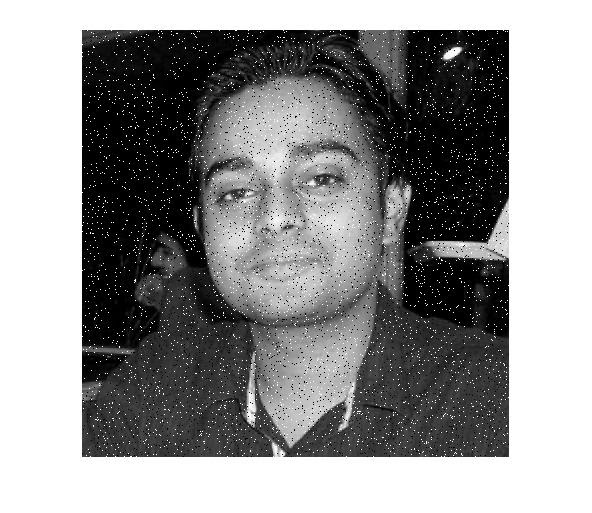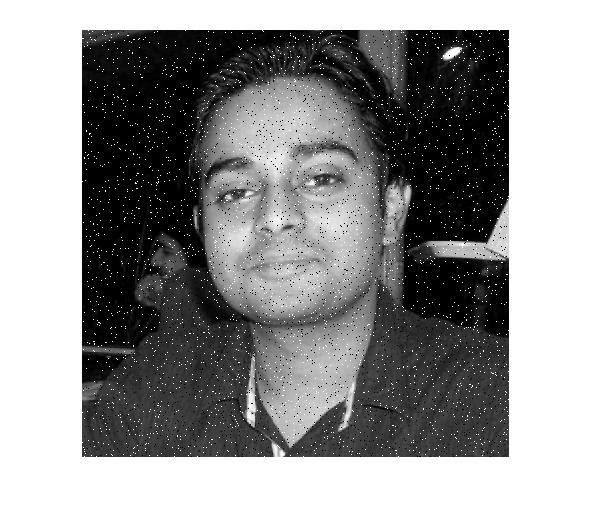# Adding Salt & Pepper Noise To An Image Using MATLAB : Using & Without Using IMNOISE Function

What is Salt & Pepper Noise?
Its an impulsive noise, which presents itself as sparsely occurring white and black pixels over an image. Its a data drop-out noise (commonly known as intensity spikes or salt and pepper noise). The noise here is caused generally by errors in the data transmission. Remember the snow like video seen on old CRT television sets, when the channel reception fails, its like the same. The corrupted pixels either set to the maximum value or to a min value (generally 240-255 for salt & 0-8 for pepper noise). Because of their color & appearance in the image they are named like that.
In worst case of transmission, where the data becomes completely corrupted, single pixels are set alternatively to zero or to the maximum value, giving the image a 'salt and pepper' like appearance. The noise is usually quantified by the percentage of pixels which are corrupted.

How to add Salt & Pepper Noise in Your Image Using MATLAB
The original image we have used is this:Method 1: By using Inbuilt "imnoise" function of MATLAB

Syntax to use 'imnoise' is,
# X = imnoise(Image, 'salt & pepper', percentage_distortion)
Above function adds "salt and pepper" noise to an image named Image, where percentage_distortion is the noise density.
# This affects approximately percentage_distortion*numel(I) pixels. The default for percentage_distortion is 0.05.

Usage:
new_i=imnoise(i,'salt & pepper', 0.05); %noise added in this step for a 5% corrupted pixels
imshow(new_i)

Results:NOTE: The function imnoise will work same for Grayscale & RGB Color image same, without any modification.

Method 1: With come logic & mathematics using MATLAB

Step 1: Select a value of Black & White Pixels of your image; For Black Choose a value of 0-8 & for a white one choose a value of 240-255. (These value will give you the appearance of salt and pepper spread over the image) . The default value in many cases are assumed to be 0 & 255 for Black & White pixels respectively, that particularly happens in high contrast images.

Step 2: Generate a random pixel to make it corrupt.

Step 3: Apply the logic to change the pixel value.

MATLAB Code Implementation of Adding Salt & Pepper noise without using imnoise is:

b=4;w=251;  %assuming our black pixel is having a value of '4' & the white one is '251'
img_with_noise= i;
%preserving the original image 'i' & operating on a new variable
[m,n]=size(i); %getting the size of image
x = randint(m,n,[0,255]);  % **See below note
%generating a random matrix of size mxn which is the size of image
%whose value of each element is randomly distributed
%over a range of 0 to 255
img_with_noise(x <= b) = 0;
%setting that pixel value '0' whose pixel value is below or equal '4'
%this step will add the pepper noise in the image

img_with_noise(x >=w) = 255;
%setting that pixel value to '255' whose pixel value is >= '251'
%this step will add the salt noise in the image
imshow(img_with_noise)%Show the corrupted image

Result of application of Above MATLAB code is:NOTE: * To make this applicable for even rgb images, use the commands to extract the image color planes & apply on them (For more reference Visit: Extracting Individual RGB Image Planes)

**The  % of the corrupted pixels which are either more biased towards salt noise or pepper noise can be controlled by the statement, x = randint(m,n,[0,255]); For making the resultant image more salty like, use any value less than '255', or to make it more pepperish use any value above '0'

*** The percentage of the corrupted pixels which are euqlly pepper & salt can be done by taking the value of 'b' & 'w' value as,
b= 255*percantage_noise/100 & w=255*percantage_noise/100
This will work since there are equal chance of getting each value at any pixel location in the image, ranging 0 - 255